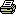# Introduction

Author: Created by: X
Website: www.createdbyx.com

This tutorial will walk you through creating a number of flies that will fallow a scent trail, that you draw on the screen using the mouse. It will be designed to show how easy it is to code AI to achieve simple pathfinding/fallowing "fly like" behavior.

What you will need

For this tutorial we will be using vb.net 2005 and the .NET framework 2.0. But if you do not have Visual Studio 2005 you can download it for free on Microsofts website. This tutorial assumes that you are familiar with the visual studio IDE, as well as the graphics objects under the System.Drawing namespace.

Getting started

To begin you must first create a new windows application, under visual studio. Second you will need to add a new code file and insert the fallowing code into it.

1. Public Module General
2. Public Function RestrictValue(ByVal V As Single, ByVal Min As Single, ByVal Max As Single) As Single
3.     If V < Min Then V = Min
4.     If V > Max Then V = Max
5.     Return V
6. End Function
7. Public Function RestrictValue(ByVal V As Integer, ByVal Min As Integer, ByVal Max As Integer) As Integer
8.     If V < Min Then V = Min
9.     If V > Max Then V = Max
10.     Return V
11. End Function
12. Public Sub Displacement(ByVal X As Single, ByVal Y As Single, ByVal Distance As Single, ByVal AngleInRadians As Single, _
13.                              ByRef NewX As Single, ByRef NewY As Single)
14.     NewX = CSng(X + (System.Math.Cos(AngleInRadians) * Distance))
15.     NewY = CSng(Y + (System.Math.Sin(AngleInRadians) * Distance))
16. End Sub
17. Public Function CircleCircle(ByVal Center1X As Single, ByVal Center1Y As Single, ByVal R1 As Single, _
18.                            ByVal Center2X As Single, ByVal Center2Y As Single, ByVal R2 As Single,_
19.                            Optional ByRef Distance As Single = 0) As Boolean
20.      Distance = CSng(Math.Sqrt((Math.Abs(Center1X - Center2X) ^ 2) + (Math.Abs(Center1Y - Center2Y) ^ 2)))
21.     Return Distance <= R1 + R2
22. End Function
23. End Module

The code provided above will be used by the application and provides simple helper functions. This code will no be covered in this tutorial as it is not relevant to the overall goal of this tutorial.

Next: Part 1 - Base Types | 1 | 2 | 3 | 4 | 5 | 6 | 7 | 8 | 9 | 10 | 11

Tutorial by:

### cbx

Date: 2006 Apr 18#### NavigatePrinter Friendly